# Poisson algebra

An algebra, usually over the field of real or complex numbers, equipped with a bilinear mapping satisfying the properties of the usual Poisson brackets of functions. Letbe an associative commutative algebra over a commutative ring(cf. Commutative algebra; Commutative ring; Associative rings and algebras). A Poisson algebra structure onis defined by an-bilinear skew-symmetric mappingsuch that

i)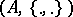is a Lie algebra over;

ii) the Leibniz rule is satisfied, namely,for all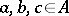. The element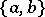is called the Poisson bracket ofand. The main example is that of the algebra of smooth functions on a Poisson manifold [a5] (cf. also Symplectic structure).

On a Poisson algebra, one can define [a12] a skew-symmetric-bilinear mapping,, which generalizes the Poisson bivector on Poisson manifolds, mapping a pair of Kähler (or formal) differentials onto the algebraitself. There exists a unique-bilinear bracket,on the-module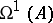of Kähler differentials satisfying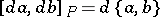and lending it the structure of a Lie–Rinehart algebra,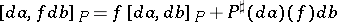, for all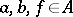. (Here,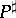is the adjoint of, mapping the Kähler differentials into the derivations of; cf. Adjoint operator.) The Poisson cohomology (cf. Cohomology) ofis then defined and, whenis projective as an-module, is equal to the cohomology of the complex of alternating-linear mappings onwith values in, with the differential [a1] defined by the Lie–Rinehart algebra structure. In the case of the algebra of functions on a differentiable manifold, the Poisson cohomology coincides with the cohomology of the complex of multivectors, with differential, whereis the Poisson bivector and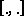is the Schouten bracket.

In a canonical ring [a4], the Poisson bracket is defined by a given mapping. Dirac structures [a13] on complexes over Lie algebras are a generalization of the Poisson algebras, adapted to the theory of infinite-dimensional Hamiltonian systems, where the ring of functions is replaced by the vector space of functionals.

In the category of-graded algebras, there are even and odd Poisson algebras, called graded Poisson algebras and Gerstenhaber algebras, respectively. Let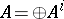be an associative, graded commutative algebra. A graded Poisson (respectively, Gerstenhaber) algebra structure onis a graded Lie algebra structure (cf. Lie algebra, graded)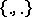(respectively, where the grading is shifted by), such that a graded version of the Leibniz rule holds: for each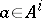,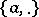is a derivation of degree(respectively,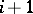) of the graded commutative algebra. Examples of Gerstenhaber algebras are: the Hochschild cohomology of an associative algebra [a2], in particular, the Schouten algebra of multivectors on a smooth manifold [a3], the exterior algebra of a Lie algebra, the algebra of differential forms on a Poisson manifold [a9], the space of sections of the exterior algebra of a Lie algebroid, the algebra of functions on an odd Poisson supermanifold of type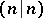[a7]. Batalin–Vil'koviskii algebras, also called BV-algebras, are exact Gerstenhaber algebras, i.e., their Lie bracket is a coboundary in the graded Hochschild cohomology of the algebra. Such structures arise on the BRST cohomology of topological field theories [a14].